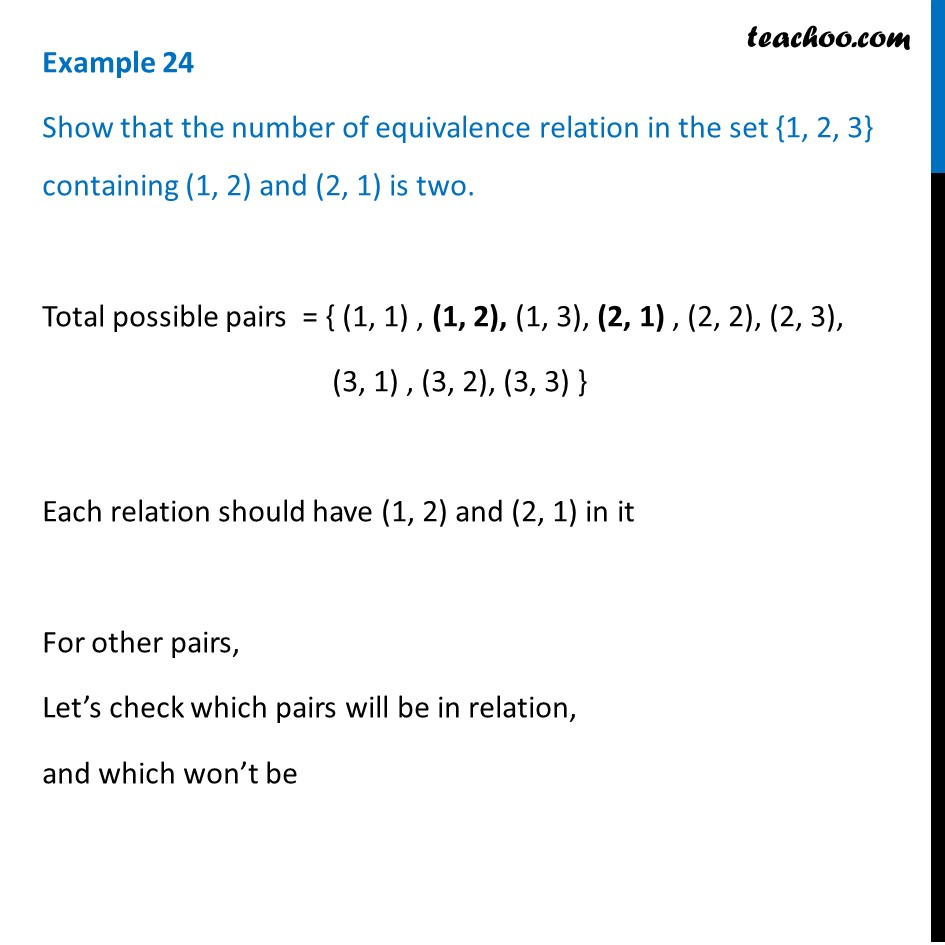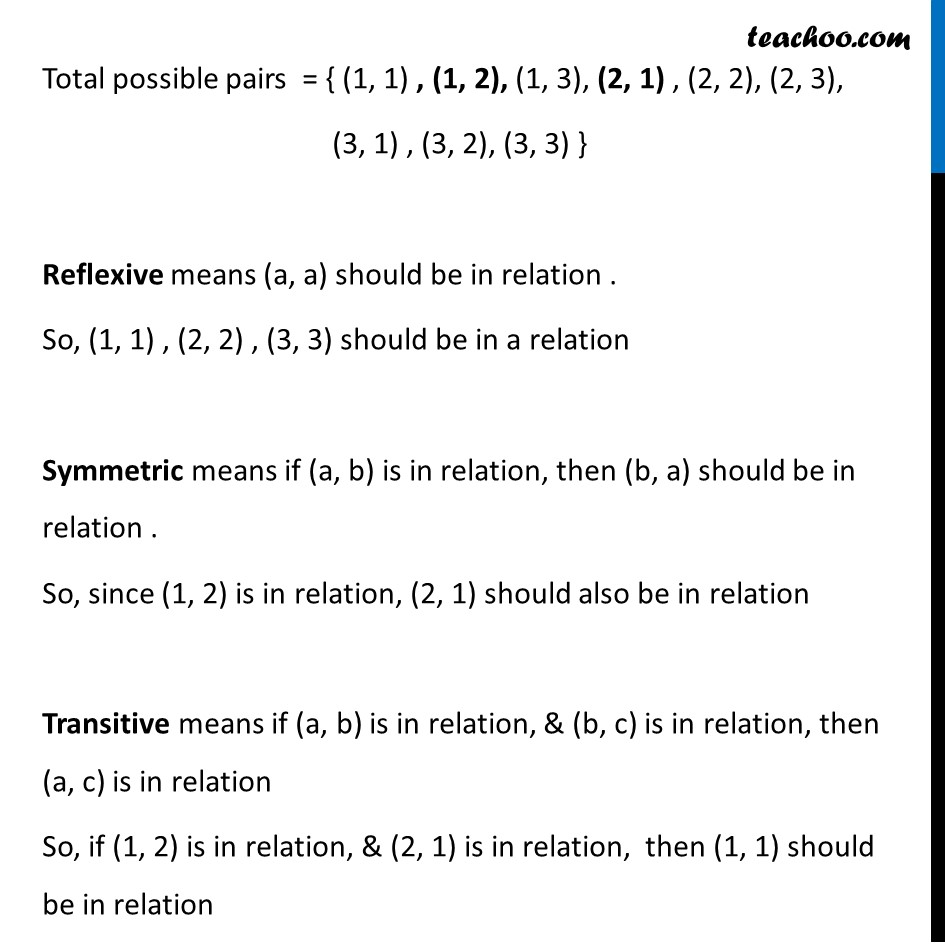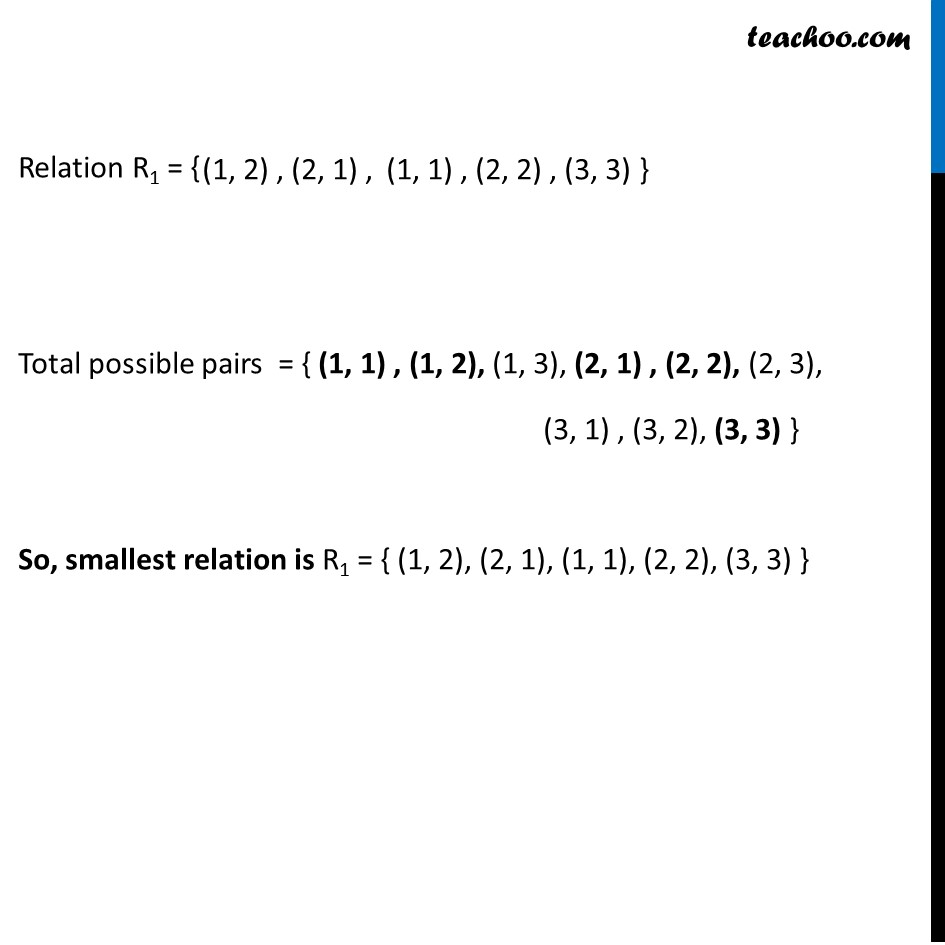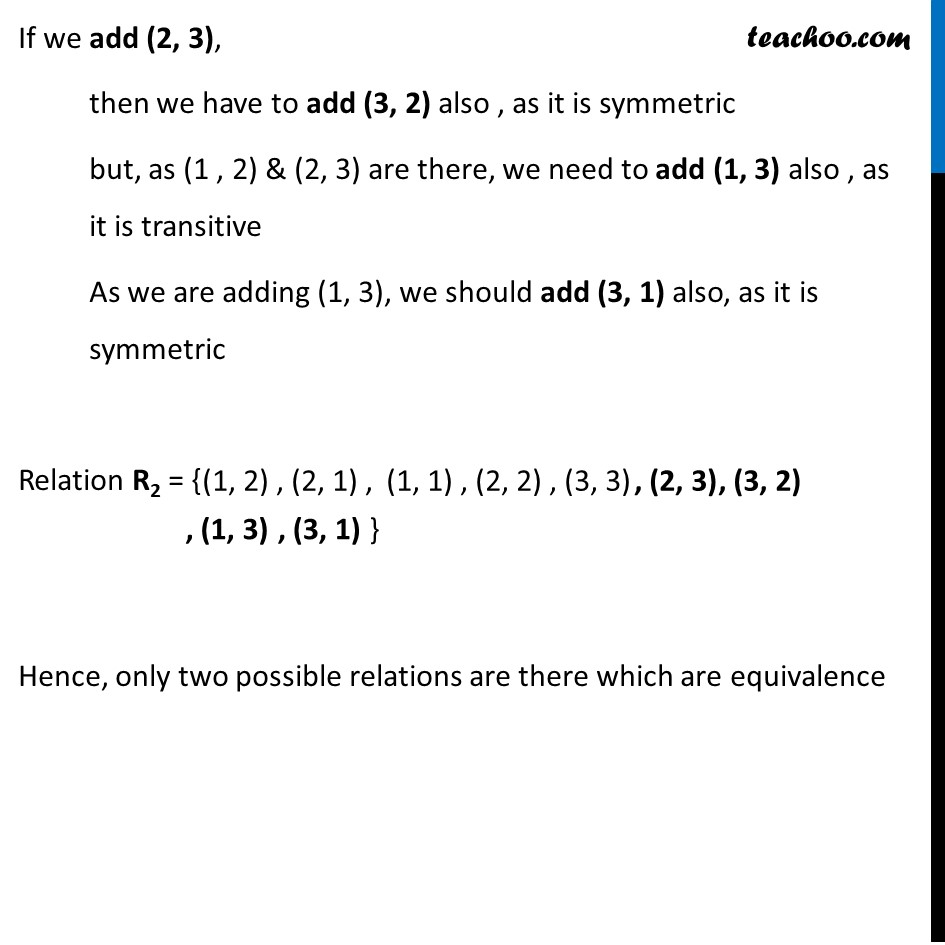Examples

Chapter 1 Class 12 Relation and Functions
Serial order wiseLearn in your speed, with individual attention - Teachoo Maths 1-on-1 Class

### Transcript

Example 24 Show that the number of equivalence relation in the set {1, 2, 3} containing (1, 2) and (2, 1) is two. Total possible pairs = { (1, 1) , (1, 2), (1, 3), (2, 1) , (2, 2), (2, 3), (3, 1) , (3, 2), (3, 3) } Each relation should have (1, 2) and (2, 1) in it For other pairs, Let’s check which pairs will be in relation, and which won’t be Total possible pairs = { (1, 1) , (1, 2), (1, 3), (2, 1) , (2, 2), (2, 3), (3, 1) , (3, 2), (3, 3) } Reflexive means (a, a) should be in relation . So, (1, 1) , (2, 2) , (3, 3) should be in a relation Symmetric means if (a, b) is in relation, then (b, a) should be in relation . So, since (1, 2) is in relation, (2, 1) should also be in relation Transitive means if (a, b) is in relation, & (b, c) is in relation, then (a, c) is in relation So, if (1, 2) is in relation, & (2, 1) is in relation, then (1, 1) should be in relation Relation R1 = { Total possible pairs = { (1, 1) , (1, 2), (1, 3), (2, 1) , (2, 2), (2, 3), (3, 1) , (3, 2), (3, 3) } So, smallest relation is R1 = { (1, 2), (2, 1), (1, 1), (2, 2), (3, 3) } If we add (2, 3), then we have to add (3, 2) also , as it is symmetric but, as (1 , 2) & (2, 3) are there, we need to add (1, 3) also , as it is transitive As we are adding (1, 3), we should add (3, 1) also, as it is symmetric Relation R2 = { Hence, only two possible relations are there which are equivalence